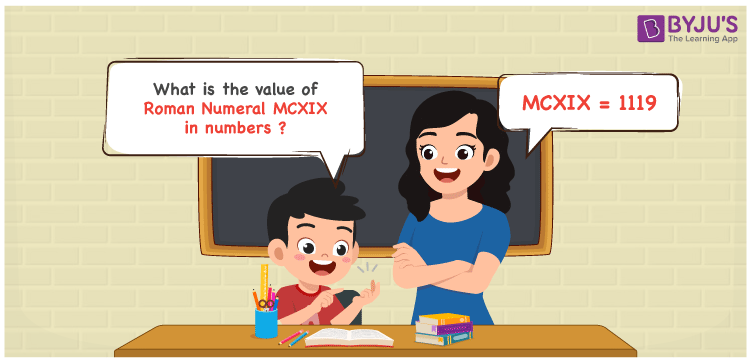Checkout JEE MAINS 2022 Question Paper Analysis : Checkout JEE MAINS 2022 Question Paper Analysis :

# MCXIX Roman Numerals

MCXIX Roman Numerals is 1119. Roman Numeral is the technique of writing numbers using the Roman alphabet. Students are recommended to access Roman Numerals 1 to 100 in PDF format to enhance effective revision of problems and score optimum marks in exams. Let us glance at how to convert MCXIX Roman Numerals in an efficient manner in this article.

 Number Roman Numeral 1119 MCXIX

## How to Write MCXIX Roman Numerals?Following methods can be used to represent the numerical value of MCXIX Roman Numerals

First Method:

• Break the Roman Numerals into single letters
• MCXIX = M + C + X + (X – I)
• Write the numerical value of each letter and add/subtract them
• MCXIX = 1000 + 100 + 10 + (10 – 1) = 1119

Second Method:

In this method, we consider the groups of Roman Numerals for addition or subtraction

• MCXIX = M + C + X + IX = 1000 + 100 + 10 + 9 = 1119
• Thus the numerical value of MCXIX Roman Numerals is 1119

## Video Lesson on Roman Numerals## Related Articles

Roman Numerals

Roman Numerals 1 to 50

Roman Numerals 1 to 1000

Roman Numerals Conversion

L Roman Numerals

Roman Numeral XXXIX

## Frequently Asked Questions on MCXIX Roman Numerals

### Write the Roman Numeral MCXIX in numbers.

Roman Numeral MCXIX in numbers is written as 1119.

### Express the sum of 1000 and 119 in Roman Numerals.

1000 + 119 = 1119. The number 1119 in Roman Numerals is written as MCXIX. Therefore the sum of 1000 and 119 in Roman Numerals is MCXIX.

### What is the difference between 1119 and 950 in Roman Numerals?

1119 – 950 = 169. The number 169 in Roman Numerals is CLXIX. Hence the difference between 1119 and 950 in Roman Numerals is CLXIX.